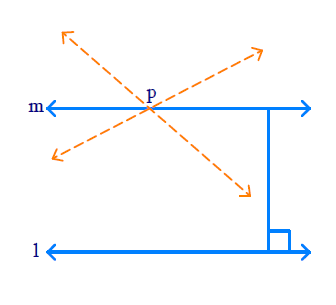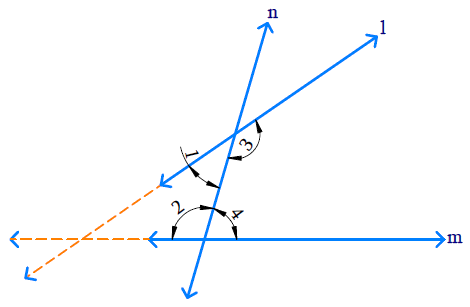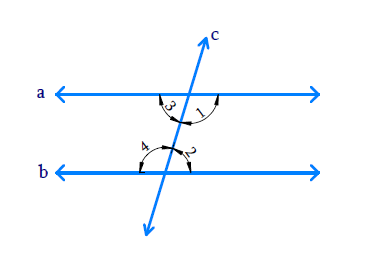# Exercise E5.2 Introduction to Euclids Geometry NCERT Solutions Class 9

Go back to  'Introduction to Euclids Geometry'

## Chapter 5 Ex.5.2 Question 1

How would you rewrite Euclid’s fifth postulate so that it would be easier to understand?

### Solution

steps:

For every line ‘$$l$$’ and for every point ‘$$P$$’ not lying on ‘$$l$$’, there exist a unique line $$‘m'$$ passing through ‘$$P$$’ and parallel to ‘$$l$$’.

This is called as ‘Playfair’s Axiom’• $$l$$ ’ is a line and ‘$$P$$ ’ is a point not lying on ’$$l$$ ’.
• We can draw infinite lines through ‘$$P$$ ’ but there is only one line unique which is parallel to ‘$$l$$ ’ and passes through ‘$$P$$ ’.
• Take any point on ‘$$l$$ ’ and draw to $$‘m'$$. Measure these distances.
• We know that it is same everywhere so these lines ‘$$l$$ ’ and $$‘m'$$ do not meet anywhere.

## Chapter 5 Ex.5.2 Question 2

Does Euclid fifth postulate imply the existence of parallel lines? Explain.

### Solution

Reasoning:

Postulate 5 : If a straight line falling on two straight lines makes the interior angles on the same side of it taken together less than two right angles, then the two straight lines, if produced indefinitely, meet on that side on which the sum of angles is less than two right angles.

Steps:

Yes

If ‘$$a$$’ & ‘$$b$$’ two straight lines which are intersected by another line ‘$$c$$’, and the sum of co-interior angles are equal to $$1800$$, then $$a\parallel b$$.According to Euclid’s 5th postulate,

\begin{align} \angle 1 + \angle 2 < 180 ^ { \circ }\end{align}  then \begin{align}\angle 3 + \angle 4 > 180 ^ { \circ } \end{align}

[The interior angles on the same side of two straight lines which are intersected by another line taken together are less than two right angles]

Producing the line ‘$$a$$’ and ‘$$b$$’ further will meet in the side of which is less than $$180 ^ {\circ}.$$

If \begin{align} \angle 1 + \angle 2 = 180 ^ { \circ } \end{align}

then  \begin{align}\angle 3 + \angle 4 = 180 ^ { \circ } \end{align}

The lines ‘$$a$$’ and ‘$$b$$’ do not meet in the side where the angle is lesser than $$180^ {\circ}$$ so they never intersect each other. Hence the two lines are said to be parallel to each other i.e. $$a\parallel b$$Related Sections
Related Sections
Instant doubt clearing with Cuemath Advanced Math Program